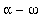Advice: Greek letters and other symbols in plots

Maple gives you control over the fonts used in axis labels, titles and other text in plots. In particular, the Symbol font can be used to obtain Greek letters and other symbols.

Here are the Symbol characters corresponding to the lowercase letters: (the html version of this page may not show the Symbol characters, depending on your browser and platform)

a b c d e f g h i j k l m n o p q r s t u v w x y z

a b c d e f g h i j k l m n o p q r s t u v w x y z

(these may not line up correctly on your screen because of differences in fonts)

the uppercase letters:

A B C D E F G H I J K L M N O P Q R S T U V W X Y Z

A B C D E F G H I J K L M N O P Q R S T U V W X Y Z

and various other keyboard characters:

~ ! @ # \$ % ^ & * ( ) _ - + = { } [ ] : ; " ' < , > . ? / | \ 1 2 3 4 5 6 7 8 9 0

~ ! @ # \$ % ^ & * ( ) _ - + = { } [ ] : ; " ' < , > . ? / | \1 2 3 4 5 6 7 8 9 0

You can use convert([n], bytes) to produce character number n as a string, where n is from

1 to 255. Here is a table of all the characters in the Symbol font, 30 per line. The font contains only characters

32 to 126 and 161 to 254 (character 32 is a blank space, and 240 may be missing on some platforms).

n

33 ! " # \$ % & ' ( ) * + , - . / 0 1 2 3 4 5 6 7 8 9 : ; `

60 < = > ? @ A B C D E F G H I J K L M N O P Q R S T U V W X Y

90 Z [ \ ] ^ _ ` a b c d e f g h i j k l m n o p q r s t u v w
120 x y z { | } ~

161 – — ˜ ™ š › œ  ž Ÿ   ¡ ¢ £ ¤ ¥ ¦ § ¨ © ª « ¬ ­ ® ¯ ° ± ² ³
180 ´ µ ¶ · ¸ ¹ º » ¼ ½ ¾ ¿ À Á Â Ã Ä Å Æ Ç È É Ê Ë Ì Í Î Ï Ð Ñ

210 Ò Ó Ô Õ Ö × Ø Ù Ú Û Ü Ý Þ ß à á â ã ä å æ ç è é ê ë ì í î ï

240 ð ñ ò ó ô õ ö ÷ ø ù ú û ü ý þ ÿ

You can copy characters from this table and paste them into your input line. The characters will look different in the Maple input line, because this is in a different font, but they can be plotted in the Symbol font.

The titlefont , labelfont , and font options apply to the plot title, labels on the axes, and text produced by textplot respectively. The axesfont option applies to the numbers beside the axis tickmarks. In the case of the Symbol font you specify this option as a list, the first item being the string SYMBOL and the second the font size in points. The default font is [HELVETICA,9] for all of these except titlefont , where the default is [TIMES,ROMAN,14] .

Thus to producecentred at the point [1,1] in a plot, you could use

> with(plots):

> textplot([1,1,`a - w`], font=[SYMBOL,12]);

Of course, you would hardly ever use this alone: instead you could combine one or more textplot results with other plots using display .

Interesting effects can be produced by combining symbols if they can be placed correctly.

For example:

> p1:= textplot([1,1.5,convert(,bytes)], font=[SYMBOL,15]):
p2:= textplot([1,1.3,convert(,bytes)], font=[SYMBOL,15]):
p3:= textplot([1.25, 1.4, `x dx`], font = [TIMES,ROMAN,15]):
p4:= textplot([1.2, 1.5, `2`], font = [TIMES, ROMAN, 12]):
p5:= plot(x^3/3, x=0..2):
display({p1,p2,p3,p4,p5});

However, the alignment of the parts of the formula changes if the size of the plot changes, and is likely to be quite different in printed output than on the screen, so this is not a satisfactory way to obtain "typeset" mathematical formulas in plots.

In the case of axes, note that a plot can only have one set of axis labels, which must all be in the same font (selected by the labelfont option). Thus you could not use the Symbol font for one axis and Helvetica for the other. What you could do, however, if using normal axes (rather than boxed or framed), is specify one of the axis labels as the empty string `` and use textplot to produce the label instead. This can't be done for boxed or framed axes, because the labels would be outside of the plotting region. For example:

> display({ plot(x^2, x = -1 .. 1, labels=[x,``],axes=normal),
textplot([-.1,.5,'a'],font=[SYMBOL,10])});

The same technique can be used to change the position of axis labels, which often interfere with tickmark labels.